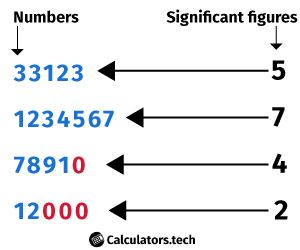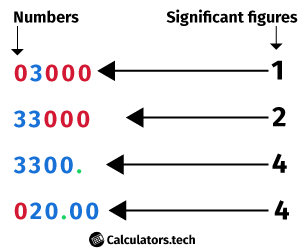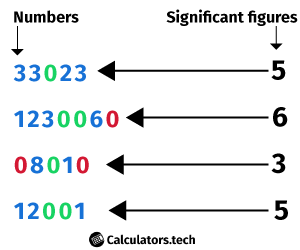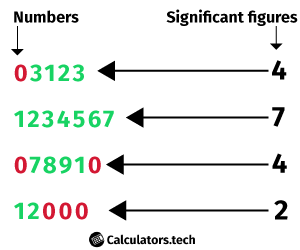# sig fig calculator

Enter Information

#### RESULTS

Fill the calculator form and click on Calculate button to get result here

Get sig fig calculator For Your Website

Our significant figures calculator converts a given number into a new number with the desired amount of significant figures and solves expressions with sig figs.
You can use this tool for your academic assignments as well as for professional fields where you get to do a lot of work with numbers.

## Significant figures definition

Sig figs are all the digits that are additional to the magnitude of a number. To avert repetitive figures that are not significant, you can round the given number.

However, you have to be very careful lest you end up losing precision while rounding. Most of the time, rounding numbers are meant for simplicity only.

## Sig Fig Rules

To determine which of the numbers are significant and which ones are not, you can use the rules of the Significant figure listed below:### The ‘0’ to the left of the decimal value less than 1 is not to be considered significant.

leading zeros which occurs before first decimal number are not considered as a significant figures.

For Example:

1) 03000 ➡ It has only one significant number

2) 020.00 ➡ it has 4 significant numbers### All of the trailing zeros that are place-holders, are not to be considered significant except when the decimal point is specified.

In many contexts, it is believed that trailing zeros are only displayed if they are significant: for instance, if a gauging that is precise to four decimal places (0.0001) were to be given as 12.23, then it would usually be mistaken to show that just the two of decimal places of precision are available.
However, when the result is described as 12.2300, it makes it clear that the value is precise to exactly four decimal places (in this case, 6 sig figs).### Zeros that occur between non-zero digits are significant.

let's find out how many sig figs are in 1101

according to the rule "All non-zero numbers are considered as the significant numbers" there are 3 sig figs. numbers when we combine both rules then we will get the correct answer which is 4.### All non-zero number values are significant.

it is very simple rules that all numbers from 1-9 considered as a significant digit. This is a very straight rule zero (0) is not included.

for example ( 011234567890 )

in the above example, there are 11 digits but have only 9 significant numbers.

### Sig Fig rules for Addition or Subtraction:

The last number reserved is set by the first doubtful number for adding and subtracting sig figs.

### Sig Fig rules for Multiplication or Division:

The answer contains no more significant numbers than the least accurately known digit for multiplying and dividing significant figures.

If a number has more digits than the required number of significant digits, the number can be rounded. For instance, 434,500 is 435,000 to three significant digits.

Zeroes at the end of the numbers that are not significant, should not be removed if you don’t round, as removal will affect the value of the number. In the above example, you can’t remove 000 in 435,000 except when you change the number into some sort of scientific notation.

## Significant figures operations

There are supplementary rules regarding the operations - addition, subtraction, multiplication and division.

The outcome of an operation cannot have more significant figures than the value with the least number of significant digits. Consider one of the typical significant figures examples, when executing the operation $$13.14 + 2.82 + 1.45$$, the value with the least number of sig figs (2) is 1.45. Therefore, the result must have two significant figures too: $$13.14 + 2.82 + 1.45 = 17.41 = 17$$.

If you’re performing addition and subtraction only, it is enough to do all the calculations at once and apply the sig figs rules to the end result.

## How to round significant figures?

Rounding significant figures comes into play when you go for mixed calculations - addition/subtraction and multiplication/division - you need to round the value for each step of calculations to the correct number of significant figures.

For instance, to calculate $$13.14 + 2.82 \times 2.5$$, after first step you’d obtain the following result: $$13.14 + 7.05$$. Then, you have to round the result of multiplication to 2 significant figures, obtaining $$13.14 + 7.05$$ Now, just add the numbers and leave two significant figures, attaining the result of $$13.14 + 7.05 = 20.19 = 20$$.

## Rounding Rules

• When rounding off numbers to a certain value of significant figures, do so to the closest value.
Example 1:
$$\mathrm{Round to 3 significant figures:} 2.3578 \times 102$$
$$\mathrm{Answer:} 2.36 \times 104$$
Example:2:
$$\mathrm{ Round to 2 significant figures:} 1.534 \times 105$$
$$\mathrm{Answer:} 1.5 \times103$$
• What to do if you encounter a 5?
There is an arbitrary approach to that: If the figure before 5 is odd, round up. If the figure before 5 is even, then just let it be. The argument for this is that in the path of sequences of many calculations, any errors in rounding would be averaged out.

## How to use the significant digits calculator

Our sig figs calculator has two functions - it executes arithmetic operations on different numbers (for instance $$4.18 / 2.33$$ or simply rounds a digit to the desired number of a significant figure.

Following the rules noted above, we can determine significant figures by hand or by using a sig fig counter. Consider that we have the number $$0.004672$$ and want 2 significant figures. The trailing zeros are placeholders, so we do not count them. Second, we round $$4672$$ to 2 digits and that leaves us with $$0.0047$$.

Now, we’d consider a value that is not a decimal. Suppose we want 4,454,689 to 4 significant figures. We simply round the entire number to the nearest thousand, giving us $$4,455,000$$.

Coming to the main task, to work your way around with sig figs with our adding/multiplying significant figure calculator, just enter the number or the expression and the round to significant figure and that is it.

This sigfig calculator is free to use and doesn’t require any subscription whatsoever.

## Accuracy and Precision in measurements

Traditionally, in different technical domains, "accuracy" refers to the close proximity of a given measured value to its true value; "precision" on the other hand, is the stability of that measurement when repeated many times for a generalization.

Hoping to reflect the way the term "accuracy" is actually used in the scientific community, a recently developed standard ISO 5725 is brought to use. It retains the same definition of precision but describes the term "trueness" as the proximity of a given measured value to its true value and employs the term of "accuracy" as the blend of trueness and precision.

In either case though, the number of sig figs fairly relates to precision and not to either use of the word ‘accuracy’ or to the modern concept of trueness.

Precise values, including defined numbers such as conversion factors and 'pure' numbers, do not affect the precision of estimation. They can be treated as though they had an infinite number of significant figs.

For example, when using the speed conversion, you have to multiply the value in m/s by 3.6 if you want to attain the value in kilometers/hour. The number of significant figs is still calculated by the accuracy of the initial speed value in m/s - for instance, $$12.56 \times 3.6 = 45.21$$.

## Application in computers

Computer representations of floating points commonly employ a type of rounding to significant digits, of course with binary numbers. The number of true significant figures is very intimately related to the idea of relative error (which has the benefit of being a more accurate gauge of precision and is sovereign of the radix, also called ‘the base,’ of the number system employed.

1. Wikipedia - Significant figures
2. Chemistry in the Community; Kendall-Hunt: Dubuque, IA 1988
3. Engelbrecht, Nancy; et al. (1990). "Rounding Decimal Numbers to a Designated Precision" (PDF). Washington, D.C.: U.S. Department of Education.
Other Languages
Related Calculators